# 3.6 Absolute value functions  (Page 3/3)

 Page 3 / 3

Access these online resources for additional instruction and practice with absolute value.

## Key concepts

• Applied problems, such as ranges of possible values, can also be solved using the absolute value function. See [link] .
• The graph of the absolute value function resembles a letter V. It has a corner point at which the graph changes direction. See [link] .
• In an absolute value equation, an unknown variable is the input of an absolute value function.
• If the absolute value of an expression is set equal to a positive number, expect two solutions for the unknown variable. See [link] .

## Verbal

How do you solve an absolute value equation?

Isolate the absolute value term so that the equation is of the form $\text{\hspace{0.17em}}|A|=B.\text{\hspace{0.17em}}$ Form one equation by setting the expression inside the absolute value symbol, $\text{\hspace{0.17em}}A,\text{\hspace{0.17em}}$ equal to the expression on the other side of the equation, $\text{\hspace{0.17em}}B.\text{\hspace{0.17em}}$ Form a second equation by setting $\text{\hspace{0.17em}}A\text{\hspace{0.17em}}$ equal to the opposite of the expression on the other side of the equation, $\text{\hspace{0.17em}}-B.\text{\hspace{0.17em}}$ Solve each equation for the variable.

How can you tell whether an absolute value function has two x -intercepts without graphing the function?

When solving an absolute value function, the isolated absolute value term is equal to a negative number. What does that tell you about the graph of the absolute value function?

The graph of the absolute value function does not cross the $\text{\hspace{0.17em}}x$ -axis, so the graph is either completely above or completely below the $\text{\hspace{0.17em}}x$ -axis.

How can you use the graph of an absolute value function to determine the x -values for which the function values are negative?

## Algebraic

Describe all numbers $\text{\hspace{0.17em}}x\text{\hspace{0.17em}}$ that are at a distance of 4 from the number 8. Express this set of numbers using absolute value notation.

Describe all numbers $\text{\hspace{0.17em}}x\text{\hspace{0.17em}}$ that are at a distance of $\text{\hspace{0.17em}}\frac{1}{2}\text{\hspace{0.17em}}$ from the number −4. Express this set of numbers using absolute value notation.

$\text{\hspace{0.17em}}|x+4|=\frac{1}{2}\text{\hspace{0.17em}}$

Describe the situation in which the distance that point $\text{\hspace{0.17em}}x\text{\hspace{0.17em}}$ is from 10 is at least 15 units. Express this set of numbers using absolute value notation.

Find all function values $\text{\hspace{0.17em}}f\left(x\right)\text{\hspace{0.17em}}$ such that the distance from $\text{\hspace{0.17em}}f\left(x\right)\text{\hspace{0.17em}}$ to the value 8 is less than 0.03 units. Express this set of numbers using absolute value notation.

$|f\left(x\right)-8|<0.03$

For the following exercises, find the x - and y -intercepts of the graphs of each function.

$f\left(x\right)=4|x-3|+4$

$f\left(x\right)=-3|x-2|-1$

$\left(0,-7\right);\text{\hspace{0.17em}}$ no $\text{\hspace{0.17em}}x$ -intercepts

$f\left(x\right)=-2|x+1|+6$

$f\left(x\right)=-5|x+2|+15$

$\left(0,\text{\hspace{0.17em}}5\right),\left(1,0\right),\left(-5,0\right)$

$f\left(x\right)=2|x-1|-6$

$\left(0,-4\right),\left(4,0\right),\left(-2,0\right)$

$f\left(x\right)=|-2x+1|-13$

$\left(0,-12\right),\left(-6,0\right),\left(7,0\right)$

$f\left(x\right)=-|x-9|+16$

$\left(0,7\right),\left(25,0\right),\left(-7,0\right)$

## Graphical

For the following exercises, graph the absolute value function. Plot at least five points by hand for each graph.

$y=|x-1|$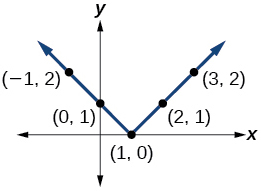$y=|x+1|$

$y=|x|+1$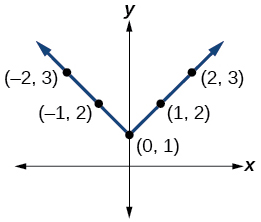For the following exercises, graph the given functions by hand.

$y=|x|-2$

$y=-|x|$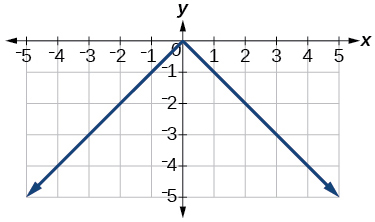$y=-|x|-2$

$y=-|x-3|-2$$f\left(x\right)=-|x-1|-2$

$f\left(x\right)=-|x+3|+4$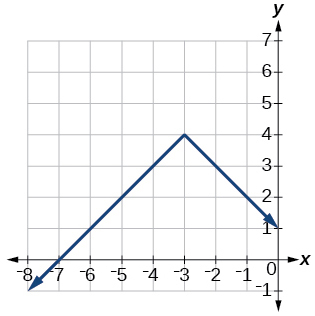$f\left(x\right)=2|x+3|+1$

$f\left(x\right)=3|x-2|+3$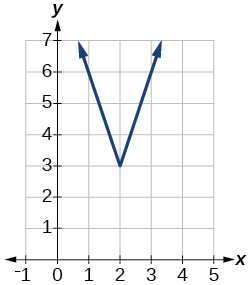$f\left(x\right)=|2x-4|-3$

$f\left(x\right)=|3x+9|+2$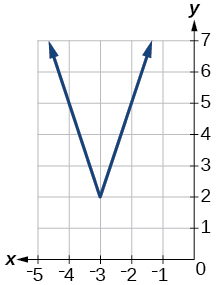$f\left(x\right)=-|x-1|-3$

$f\left(x\right)=-|x+4|-3$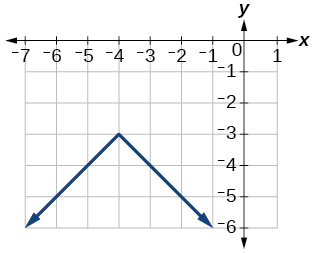$f\left(x\right)=\frac{1}{2}|x+4|-3$

## Technology

Use a graphing utility to graph $f\left(x\right)=10|x-2|$ on the viewing window $\left[0,4\right].$ Identify the corresponding range. Show the graph.

range: $\text{\hspace{0.17em}}\left[0,20\right]$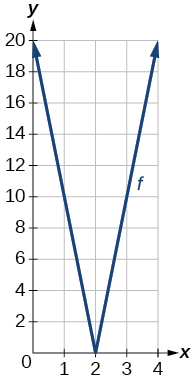Use a graphing utility to graph $\text{\hspace{0.17em}}f\left(x\right)=-100|x|+100\text{\hspace{0.17em}}$ on the viewing window $\text{\hspace{0.17em}}\left[-5,5\right].\text{\hspace{0.17em}}$ Identify the corresponding range. Show the graph.

For the following exercises, graph each function using a graphing utility. Specify the viewing window.

$f\left(x\right)=-0.1|0.1\left(0.2-x\right)|+0.3$

$x\text{-}$ intercepts: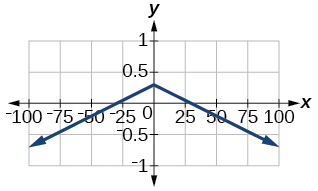$f\left(x\right)=4×{10}^{9}|x-\left(5×{10}^{9}\right)|+2×{10}^{9}$

## Extensions

For the following exercises, solve the inequality.

If possible, find all values of $a$ such that there are no $x\text{-}$ intercepts for $f\left(x\right)=2|x+1|+a.$

If possible, find all values of $\text{\hspace{0.17em}}a\text{\hspace{0.17em}}$ such that there are no $\text{\hspace{0.17em}}y$ -intercepts for $\text{\hspace{0.17em}}f\left(x\right)=2|x+1|+a.$

There is no solution for $\text{\hspace{0.17em}}a\text{\hspace{0.17em}}$ that will keep the function from having a $\text{\hspace{0.17em}}y$ -intercept. The absolute value function always crosses the $\text{\hspace{0.17em}}y$ -intercept when $\text{\hspace{0.17em}}x=0.$

## Real-world applications

Cities A and B are on the same east-west line. Assume that city A is located at the origin. If the distance from city A to city B is at least 100 miles and $\text{\hspace{0.17em}}x\text{\hspace{0.17em}}$ represents the distance from city B to city A, express this using absolute value notation.

The true proportion $\text{\hspace{0.17em}}p\text{\hspace{0.17em}}$ of people who give a favorable rating to Congress is 8% with a margin of error of 1.5%. Describe this statement using an absolute value equation.

$|p-0.08|\le 0.015$

Students who score within 18 points of the number 82 will pass a particular test. Write this statement using absolute value notation and use the variable $\text{\hspace{0.17em}}x\text{\hspace{0.17em}}$ for the score.

A machinist must produce a bearing that is within 0.01 inches of the correct diameter of 5.0 inches. Using $\text{\hspace{0.17em}}x\text{\hspace{0.17em}}$ as the diameter of the bearing, write this statement using absolute value notation.

$|x-5.0|\le 0.01$

The tolerance for a ball bearing is 0.01. If the true diameter of the bearing is to be 2.0 inches and the measured value of the diameter is $\text{\hspace{0.17em}}x\text{\hspace{0.17em}}$ inches, express the tolerance using absolute value notation.

#### Questions & Answers

A laser rangefinder is locked on a comet approaching Earth. The distance g(x), in kilometers, of the comet after x days, for x in the interval 0 to 30 days, is given by g(x)=250,000csc(π30x). Graph g(x) on the interval [0, 35]. Evaluate g(5)  and interpret the information. What is the minimum distance between the comet and Earth? When does this occur? To which constant in the equation does this correspond? Find and discuss the meaning of any vertical asymptotes.
Kaitlyn Reply
The sequence is {1,-1,1-1.....} has
amit Reply
circular region of radious
Kainat Reply
how can we solve this problem
Joel Reply
Sin(A+B) = sinBcosA+cosBsinA
Eseka Reply
Prove it
Eseka
Please prove it
Eseka
hi
Joel
June needs 45 gallons of punch. 2 different coolers. Bigger cooler is 5 times as large as smaller cooler. How many gallons in each cooler?
Arleathia Reply
7.5 and 37.5
Nando
find the sum of 28th term of the AP 3+10+17+---------
Prince Reply
I think you should say "28 terms" instead of "28th term"
Vedant
the 28th term is 175
Nando
192
Kenneth
if sequence sn is a such that sn>0 for all n and lim sn=0than prove that lim (s1 s2............ sn) ke hole power n =n
SANDESH Reply
write down the polynomial function with root 1/3,2,-3 with solution
Gift Reply
if A and B are subspaces of V prove that (A+B)/B=A/(A-B)
Pream Reply
write down the value of each of the following in surd form a)cos(-65°) b)sin(-180°)c)tan(225°)d)tan(135°)
Oroke Reply
Prove that (sinA/1-cosA - 1-cosA/sinA) (cosA/1-sinA - 1-sinA/cosA) = 4
kiruba Reply
what is the answer to dividing negative index
Morosi Reply
In a triangle ABC prove that. (b+c)cosA+(c+a)cosB+(a+b)cisC=a+b+c.
Shivam Reply
give me the waec 2019 questions
Aaron Reply

### Read also:

#### Get the best Algebra and trigonometry course in your pocket!

Source:  OpenStax, Algebra and trigonometry. OpenStax CNX. Nov 14, 2016 Download for free at https://legacy.cnx.org/content/col11758/1.6
Google Play and the Google Play logo are trademarks of Google Inc.

Notification Switch

Would you like to follow the 'Algebra and trigonometry' conversation and receive update notifications?ByByByBy RhodesBy